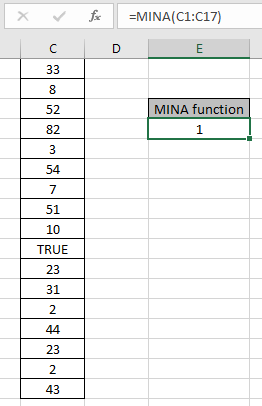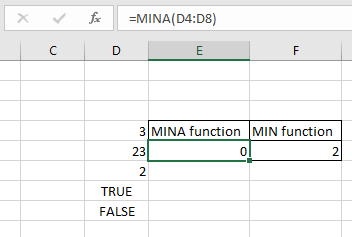# How to use the MINA function in ExcelIn this article, we will learn how to use MINA Function in Excel. MINA function is a mathematical function which returns the numerical smallest value from an array of numbers given in as input. The MINA function considers logic values also whereas MIN function ignores. MINA function given a list of values as arguments returns the minimum numeric value in the cell.
Syntax:

=MINA(number1,number2,..)

number : number or array given as cell reference

Example:

All of these might be confusing to understand. So, let's test this formula via running it on the example shown below. Here we have some numbers to test the MINA function in Excel.

Use the formula:

=MINA(C1:C17)
=MINA(C1:C17)

Here the array of values is given as cell reference. You can also input values as numbers.Here the function ignores blank cells, TRUE logic value is considered as 1. The function returns 1 as numerically the smallest value from the array instead of 2 which is numerically smallest.

Now consider one more example of MINA function:
Here we take numbers and Logic values in array and take the respective from both MINA and MIN function2 is the numerically the smallest value so the MIN function ignores logic values and returns -3 but MINA considers TRUE as 1 and FALSE as 0 numeric value and returns 0 as result.

Here are some observational notes shown below.

Notes:

1. The formula only works with numbers and text.
2. The MINA function considers logic values TRUE as 1 and FALSE as 0 whereas MIN function ignores.
3. The function takes 0 if no number is given in as input.

Hope this article about How to use the MINA function in Excel is explanatory. Find more articles on mathematical formulas here. If you liked our blogs, share it with your fristarts on Facebook. And also you can follow us on Twitter and Facebook. We would love to hear from you, do let us know how we can improve, complement or innovate our work and make it better for you. Write to us at info@exceltip.com.

Related Articles

How to use the MAX function in Excel | returns the numerical largest value from the given array using the MAX function in Excel

How to use the MIN function in Excel | returns the numerical smallest value from the given array using the MIN function in Excel

How to use the LARGE function in Excel | returns the numerical largest nth value from the given array using the LARGE function in Excel

How to use the ISEVEN function in Excel | returns the TRUE logic value if the number is MAX using the ISEVEN function in Excel.

How to use the ISODD function in Excel | returns the TRUE logic value if the number is ODD using the ISODD function in Excel.

Popular Articles:

50 Excel Shortcuts to Increase Your Productivity | Get faster at your task. These 50 shortcuts will make you work even faster on Excel.

The VLOOKUP Function in Excel | This is one of the most used and popular functions of excel that is used to lookup value from different ranges and sheets.

COUNTIF in Excel 2016 | Count values with conditions using this amazing function. You don't need to filter your data to count specific values. Countif function is essential to prepare your dashboard.

How to Use SUMIF Function in Excel | This is another dashboard essential function. This helps you sum up values on specific conditions.

Terms and Conditions of use

The applications/code on this site are distributed as is and without warranties or liability. In no event shall the owner of the copyrights, or the authors of the applications/code be liable for any loss of profit, any problems or any damage resulting from the use or evaluation of the applications/code.